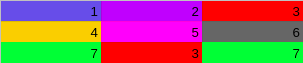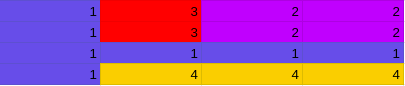0

# Stamp Box

Difficulty: MEDIUMContributed By
Shrey Pansuria
Avg. time to solve
25 min
Success Rate
75%

Problem Statement

#### Ninja is supposed to draw the given design under the given conditions:

``````1. Ninja can use one color at a time.
2. Ninja can’t use one color more than once.
3. The stamp box is in the form of a rectangle. So, Ninja can only draw rectangles(or squares) by adjusting the dimensions of the stamp box.
4. Once Ninja prints any color using the stamp box, the old color won’t be visible and only the new color will be visible.
``````

#### Can you help Ninja to find if it is possible to print the design or not?

##### Input Format:
``````The first line of input contains an integer ‘T’, denoting the number of test cases. The test cases follow.

The first line contains two space-separated integers ‘N’ and ‘M’, which denotes the number of rows and columns in the matrix ‘MAT’.

The next ‘N’ lines contain ‘M’ space-separated integers denoting the colors at that point.
``````
##### Output Format:
``````For each test case, print 1 if it is possible to draw the given design. Otherwise, print 0.

Print the output of each test case in a separate line.
``````
##### Note:
``````You are not required to print the expected output, it has already been taken care of. Just implement the function.
``````
##### Constraints:
``````1 <= T <= 50
1 <= N <= 100
1 <= M <= 100
1 <= MAT[i][j] <= 50

Where 'MAT[i][j]' is the color in the coordinate (i, j).

Time Limit: 1 sec
``````
##### Sample Input 1:
``````2
3 3
1 2 3
4 5 6
7 3 7
4 4
1 3 2 2
1 3 2 2
1 1 1 1
1 4 4 4
``````
##### Sample Output 1:
``````0
1
``````
##### Explanation For Sample Input 1:
``````In the first test case, there are 7 colors in a matrix of size 3X3 as shown in the figure.
````````````To create the given design, we need to reuse one of the two colors - 3 or 7. Otherwise, it is not possible to make the given design under the given conditions. So, the output is 0.

In the second test case, there are 4 colors in a matrix of size 4X3 as shown in the figure.
````````````To create the given design,
One of the options to make the given design is:
Make the stamp box of size 3X3 and print color 1 in all the boxes.
Make the stamp box of size 2X2 and then print color 2 in the top right corner.
Make the stamp box of size 2X1 and then print color 3 beside color 2.
Make the stamp box of size 1X3 and then print color 1 behind color 2.
Hence, it is possible to make this design. So, the output is 1.
``````
##### Sample Input 2:
``````2
4 3
1 2 1
1 3 1
1 1 3
1 1 1
2 2
1 2
3 4
``````
##### Sample Output 2:
``````0
1
``````
##### Explanation For Sample Input 2:
``````In the first test case, it is possible to draw the given design. Thus, we will print 1 as output.
In the second test case, it is impossible to draw the design. So, we will print 0.
``````Console